# Test: Recursion- 2

## 20 Questions MCQ Test GATE Computer Science Engineering(CSE) 2023 Mock Test Series | Test: Recursion- 2

Description
Attempt Test: Recursion- 2 | 20 questions in 60 minutes | Mock test for Computer Science Engineering (CSE) preparation | Free important questions MCQ to study GATE Computer Science Engineering(CSE) 2023 Mock Test Series for Computer Science Engineering (CSE) Exam | Download free PDF with solutions
QUESTION: 1

### Predict output of following program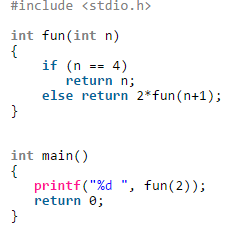Solution:

Fun(2)
|
2*fun(3)
|
2*fun(4)
|
4 After tree evaluation we get 16
So, C is the correct answer

QUESTION: 2

### Consider the following recursive function fun(x, y). What is the value of fun(4, 3)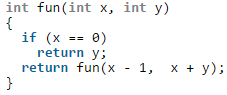Solution:

The function fun() calculates and returns ((1 + 2 … + x-1 + x) +y) which is x(x+1)/2 + y.

QUESTION: 3

### What does the following function print for n = 25?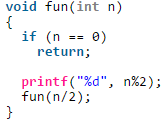Solution:

The function mainly prints binary representation in reverse order.

QUESTION: 4

What does the following function do?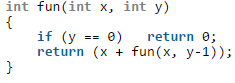Solution:

The function adds x to itself y times which is x*y.

QUESTION: 5

What does fun2() do in general?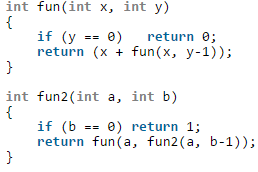Solution:

The function multiplies x to itself y times which is xy.

QUESTION: 6

Output of following program?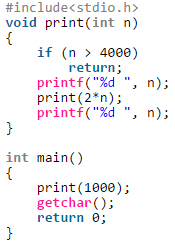Solution:
QUESTION: 7

What does the following function do?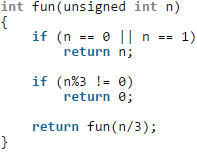Solution:
QUESTION: 8

Predict the output of following program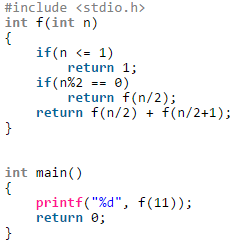Solution:
QUESTION: 9

Consider the following recursive C function that takes two arguments

unsigned int foo(unsigned int n, unsigned int r){

if(n >0) return (n%r + foo (n/r, r));

else return 0;

}

Q.
What is the return value of the function foo when it is called as foo(345, 10) ?

Solution:

The call foo(345, 10) returns sum of decimal digits (because r is 10) in the number n. Sum of digits for 345 is 3 + 4 + 5 = 12.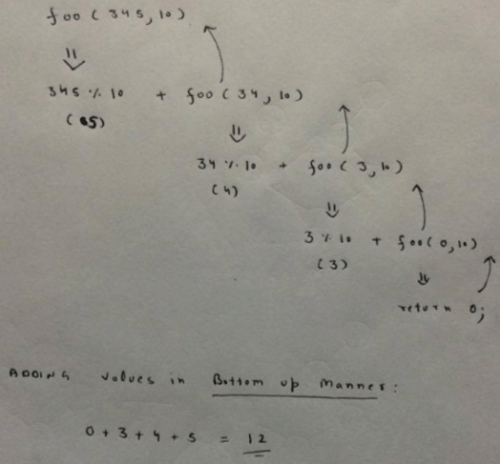QUESTION: 10

Consider the same recursive C function that takes two arguments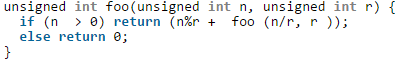Q. What is the return value of the function foo when it is called as foo(513, 2)?

Solution:

foo(513, 2) will return 1 + foo(256, 2). All subsequent recursive calls (including foo(256, 2)) will return 0 + foo(n/2, 2) except the last call foo(1, 2). The last call foo(1, 2) returns 1. So, the value returned by foo(513, 2) is 1 + 0 + 0…. + 0 + 1. The function foo(n, 2) basically returns sum of bits (or count of set bits) in the number n.

QUESTION: 11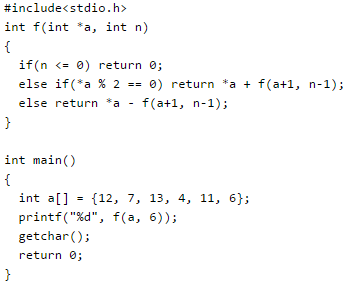Solution:

f() is a recursive function which adds f(a+1, n-1) to *a if *a is even. If *a is odd then f() subtracts f(a+1, n-1) from *a. See below recursion tree for execution of f(a, 6).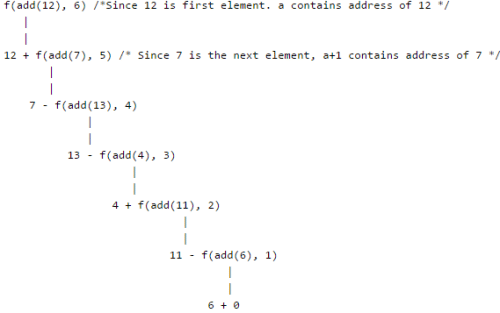So, the final returned value is 12 + (7 – (13 – (4 + (11 – (6 + 0))))) = 15

QUESTION: 12

Output of following program?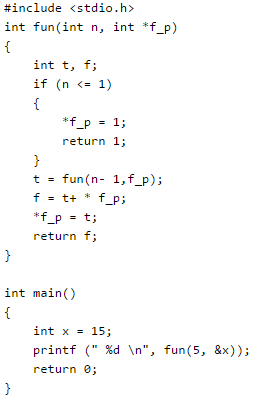Solution: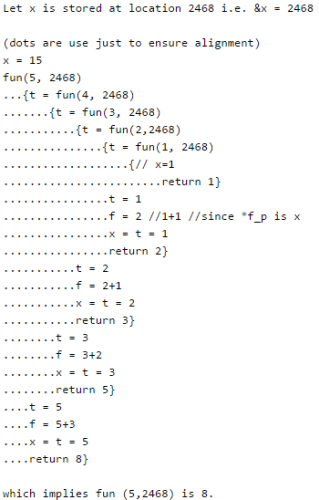QUESTION: 13

Consider the following code snippet: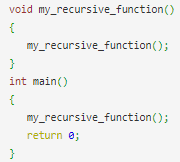What will happen when the above snippet is executed?

Solution:

Every function call is stored in the stack memory. In this case, there is no terminating condition(base case). So, my_recursive_function() will be called continuously till the stack overflows and there is no more space to store the function calls. At this point of time, the program will stop abruptly.

QUESTION: 14

Consider the C function given below.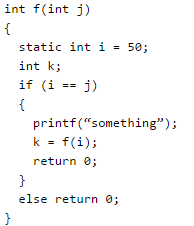Q. Which one of the following is TRUE?

Solution:

When j is 50, the function would call itself again and again as neither i nor j is changed inside the recursion.

QUESTION: 15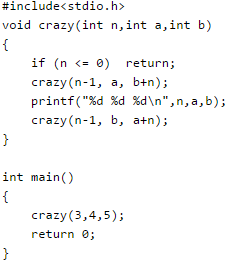Solution:
QUESTION: 16

Consider the following C function: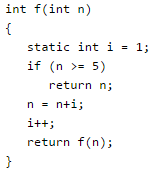The value returned by f(1) is

Solution:
QUESTION: 17

Consider the following C function.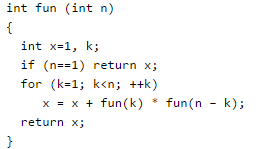Q. The return value of fun(5) is __________.

Solution: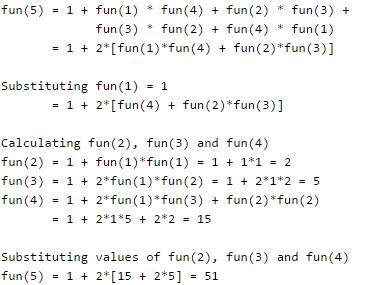QUESTION: 18

Consider the following recursive C function. If get(6) function is being called in main() then how many times will the get() function be invoked before returning to the main()?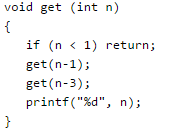Solution: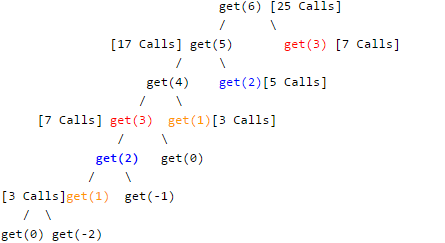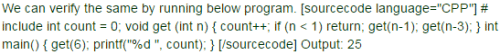QUESTION: 19

What will be the output of the following C program?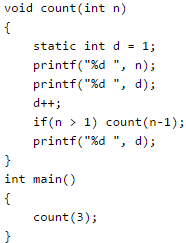Solution:

count(3) will print value of n and d. So 3 1 will be printed and d will become 2.
Then count(2) will be called. It will print value of n and d.
So 2 2 will be printed and d will become 3.
Then count(1) will be called. It will print value of n and d.
So 1 3 will be printed and d will become 4.
Now count(1) will print value of d which is 4. count(1) will finish its execution.
Then count(2) will print value of d which is 4.
Similarly, count(3) will print value of d which is 4.
So series will be A.

QUESTION: 20

The function f is defined as follows: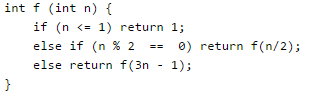Assuming that arbitrarily large integers can be passed as a parameter to the function, consider the following statements.
1. The function f terminates for finitely many different values of n ≥ 1.
ii. The function f terminates for infinitely many different values of n ≥ 1.
iii. The function f does not terminate for finitely many different values of n ≥ 1.
iv. The function f does not terminate for infinitely many different values of n ≥ 1.
Which one of the following options is true of the above?

Solution:

The function terminates for all values having a factor of 2 {(2.x)2==0}
So, (i) is false and (ii) is TRUE.
Let n = 3, it will terminate in 2nd iteration.
Let n=5, it will go like 5 - 14 - 7 - 20 - 10 - 5 – and now it will repeat.
And any number with a factor of 5 and 2, there are infinite recursions possible.
So, (iv) is TRUE and (iii) is false.Use Code STAYHOME200 and get INR 200 additional OFF Use Coupon Code